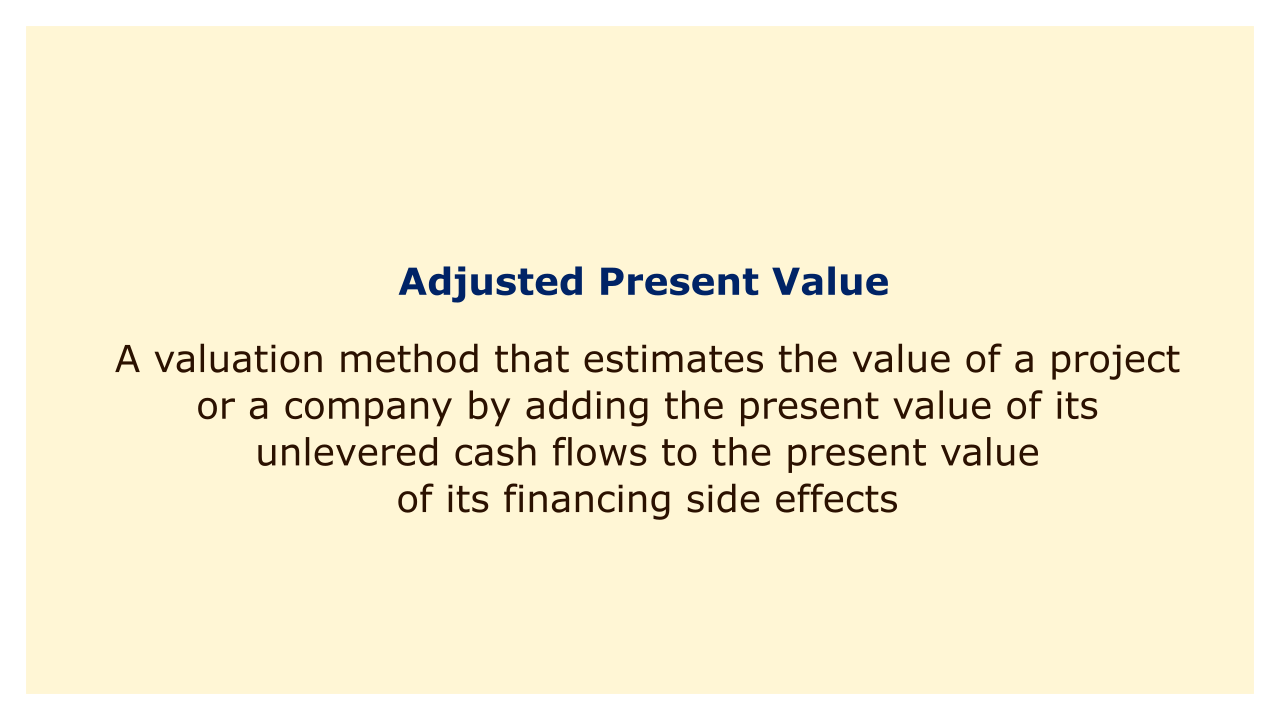Image: Moneybestpal.com

### Adjusted Present Value (APV) is a valuation method that estimates the value of a project or a company by adding the present value of its unlevered cash flows to the present value of its financing side effects.

Unlevered cash flows are the cash flows a project or business would produce if it had no debt. The advantages or expenses related to using debt for financing, such as tax breaks, bankruptcy fees, agency costs, etc., are called financing side effects.

The fundamental benefit of APV is that it separates the value of the project or business from its capital structure, which makes it simpler to compare various financing options and determine how debt affects value. Moreover, APV provides greater modeling flexibility for complicated financing arrangements like variable debt levels, numerous sources of debt, or hybrid securities.

The fundamental drawback of APV is that it requires more inputs and assumptions than other methods of valuation, like the capital asset pricing model (CAPM) or the weighted average cost of capital (WACC) . For the unlevered cash flows and the financing side effects, APV also depends on establishing the right discount rate, which can be difficult and arbitrary.

The basic formula for APV is:

APV = PV(U) + PV(F)

where:
• APV = Adjusted Present Value
• PV(U) = Present Value of Unlevered Cash Flows
• PV(F) = Present Value of Financing Side Effects

To calculate the PV(U), we need to estimate the unlevered cash flows and discount them at the unlevered cost of equity, which is the required return for an all-equity financed project or company. The unlevered cost of equity can be estimated using the CAPM as follows:

r_u = r_f + β_u * (r_m - r_f)

where:
• r_u = unlevered cost of equity
• r_f = risk-free rate
• β_u = unlevered beta
• r_m = market return

The unlevered beta measures the systematic risk of the project or company without debt. It can be derived from the levered beta, which is the observed beta of a comparable company or industry, by using the following formula:

β_u = β_l / [1 + (1 - t) * (D/E)]

where:
• β_u = unlevered beta
• β_l = levered beta
• t = corporate tax rate
• D = market value of debt
• E = market value of equity

To calculate the PV(F), we need to identify and quantify the financing side effects and discount them at their respective discount rates. The most common financing side effect is the tax shield, which is the reduction in taxes due to the interest payments on debt. The tax shield can be calculated as:

TS = t * I

where:
• TS = tax shield
• t = corporate tax rate
• I = interest payment

The present value of the tax shield depends on how we assume the debt level changes over time. There are two main approaches: constant debt and growing debt.

#### Constant debt:

This approach makes the assumption that the amount of debt will remain the same over the course of the undertaking or business. An annuity that can be discounted at the cost of debt, which is the interest rate, serves as the tax shield in this instance. The formula for the present value of the tax shield is:

PV(TS) = TS / r_d

where:
• - PV(TS) = present value of tax shield
• - TS = tax shield
• - r_d = cost of debt

#### Growing debt:

This method makes the assumption that the unlevered cash flows and debt level increase at a constant rate. The tax shield in this instance is an ever-increasing perpetuity that can be valued at the difference between the cost of debt and the growth rate. The formula for the present value of the tax shield is:

PV(TS) = TS / (r_d - g)

where:
• PV(TS) = present value of tax shield
• TS = tax shield
• r_d = cost of debt
• g = growth rate

Other financing side effects that may affect the value of a project or company include:
• Bankruptcy costs: These include the direct and indirect expenses of financial distress and default, including the cost of attorneys' fees, missed sales, decreased investment, etc. A project's or business's worth is decreased by bankruptcy expenses, which can be calculated as a percentage of the unlevered value of the project or business. The unlevered cost of equity can be used to discount the present value of bankruptcy costs.
• Agency costs: These are the expenses brought on by conflicts of interest among various stakeholders, including managers, shareholders, and creditors. Agency expenses can be calculated as a percentage of the project or company's unlevered value and lower the value of the endeavor. At the unlevered cost of equity, the present value of agency expenses can be discounted.
• Subsidies: These are the benefits or incentives offered by the government or other organizations to promote or support specific initiatives or businesses, such as grants, tax credits, guarantees, etc. A project's or business's value will increase as a result of subsidies, which can be calculated as a percentage of the unlevered value. At the unlevered cost of equity, the present value of subsidies can be discounted.
Tags